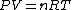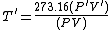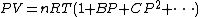# gas thermometry

Also found in: Dictionary, Thesaurus, Medical.

## Gas thermometry

A method of measuring temperatures with gas as the thermometric fluid. Gas thermometry is the primary source of information about a fundamental physical parameter, temperature, over the range from about 3 to 900 K (-454 to 1160°F).

In principle, gas thermometry consists of using the ideal gas law, Eq. (1),

(1)where P is the pressure, V the volume, n the number of moles of gas, R the molar gas constant, and T the thermodynamic temperature, to evaluate an unknown temperature by reference to the single defining temperature of the Kelvin thermodynamic temperature scale, namely the triple-point temperature of water. This reference temperature, achievable within about one part in 107 by standard laboratory practice, has the value 273.16 K (0.01°C or 32.018°F). Determination of the unknown temperature requires two sets of measurements of the pressure P and the volume V of n moles of an ideal gas. One set of measurements usually is performed while the gas is maintained at the water triple-point temperature; the second set is obtained with the gas at the unknown temperature. The unknown temperature can readily be evaluated by rewriting Eq. (1) as n = (PV)/(RT), which has the same value for both sets of measurements. The desired result is given by Eq. (2),
(2)where the primed quantities refer to the unknown temperature. In Eq. (2) the precise values of the gas constant R and of n need not be considered.

Constant-volume gas thermometry is by far the most commonly used of the gas-thermometry methods. The name is somewhat misleading because no gas bulb truly exhibits constant volume over any substantial temperature range. Several steps are involved: inserting a fixed mass of a working gas into a rigid container or bulb; determining the pressure of the gas at the triple-point temperature of water; heating or cooling the container to a new temperature whose value is to be determined; and measuring the gas pressure at the new temperature.

A typical constant-volume gas thermometer (see illustration) includes a mercury manometer, used for the measurement of pressure; a gas-bulb system; and a gas-handling system. The part-per-million accuracy achievable in manometric pressure measurements is central to the thermometric accuracy of this instrument. The entire manometer is operated in a temperature-controlled environment. The distance between the surface of the mercury in an upper cell and mercury surfaces in two lower cells is measured by the use of wrung stacks of calibrated end gage blocks. Axial holes through the gage blocks permit detection of the quality of the wringing process by measurement of the internal vacuum of the stack. The pressure exerted by the column of mercury is given by the product &rgr;gh, where &rgr; is the density of the mercury, g is the acceleration due to gravity at the manometer, and h is the height of the gage-block stack.

The gas bulb is completely enclosed by a second bulb in which a so-called counterpressure of helium gas equal to the gas-bulb pressure is maintained at all times. The counterpressure gas minimizes pressure-induced changes in the gas-bulb volume and helps to reduce contamination of the working gas from impurities in the gas-bulb thermostat.

It is possible to determine the thermodynamic temperature of a gas bulb by repeatedly adding measured quantities of a working gas to it and measuring the resulting pressures. The repeated measurements at a single temperature are known as an isotherm. The virial equation (3)

(3)can be used to obtain both the unknown gas-bulb temperature and the value of the second virial coefficient. See Virial equation

Two variations on the technique can be used, the absolute isotherm and the relative isotherm. In absolute isothermal gas thermometry, measured quantities of working gas are introduced into a gas bulb at the unknown temperature from a known volume that is maintained at 273.16 K. In the relative isotherm method, the working gas is added stepwise to a gas bulb while it is maintained at another, more convenient reference temperature. See Low-temperature thermometry, Physical measurement, Temperature measurement

McGraw-Hill Concise Encyclopedia of Physics. © 2002 by The McGraw-Hill Companies, Inc.

## gas thermometry

[¦gas thər′mäm·ə·trē]
(engineering)
Measurement of temperatures with a gas thermometer; used with helium down to about 1 K.
McGraw-Hill Dictionary of Scientific & Technical Terms, 6E, Copyright © 2003 by The McGraw-Hill Companies, Inc.
Site: Follow: Share:
Open / Close